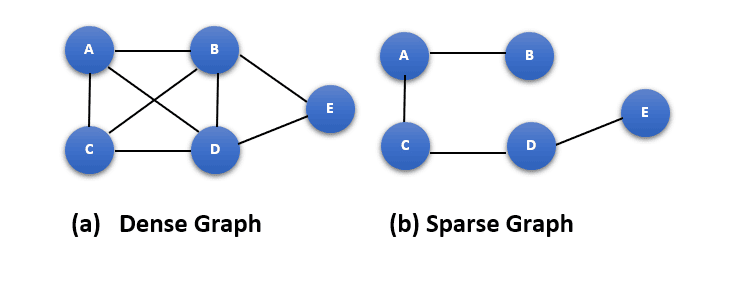## 1. Overview

In this tutorial, we’ll provide a quick introduction to the sparse coding neural networks concept.

Sparse coding, in simple words, is a machine learning approach in which a dictionary of basis functions is learned and then used to represent input as a linear combination of a minimal number of these basis functions.

Moreover, sparse coding seeks a compact, efficient data representation, which may be utilized for tasks such as denoising, compression, and classification.

## 2. Sparse Coding

In the case of sparse coding, the data is first translated into a high-dimensional feature space, where the basis functions are then employed to represent the data as a linear combination of a limited number of these basis functions.

Subject to a sparsity constraint that promotes the coefficients to be zero or nearly zero, the coefficients of linear combinations are selected to reduce the reconstruction error between the original data and the representation. So, with most of the coefficients being zero or almost zero, this sparsity constraint pushes the final representation to be a linear combination of the basis functions.

In this way, sparse coding, as previously noted, seeks to discover a usable sparse representation of any given data. Then, a sparse code will be used to encode data:

• To learn the sparse representation, the sparse coding requires input data
• This is incredibly helpful since you can utilize unsupervised learning to apply it straight to any data
• Without sacrificing any information, it will automatically find the representation

Sparse coding techniques aim to meet the following two constraints simultaneously:

• It’ll try to learn how to create a useful sparse representation as a vectorfor a given data(as a vector) using an encoder matrix• It’ll try to reconstruct the datausing a decoder matrixfor each representation is given as a vector### 2.1. Mathematical Background of Sparse Coding

We assume our datasatisfies the following equation:

(1)• Sparse learning: a process that aims to train the input data andto generate a dictionary of basis functions• Sparse encodinga process that aims to learn the sparse code h using the test dataand the generated dictionary ()

The general form of the target function to optimize the dictionary learning process may be mathematically represented as follows:

(2)In the previous equation,is a constant;denotes the dimensionality of data; andmeans the k-given vector of the data. Furthermore,is the sparse representation of;is the decoder matrix (dictionary); and the coefficient of sparsity is.

The min expression in the general form gives the impression that we are attempting to nest two optimization issues. We can think of this as two separate optimization issues fighting to get the optimal compromise:

• Outer minimization: the procedure that deals with the left side of the sum process while attempting to adjustto reduce the input’s loss during the reconstruction process
• Inner minimization: the procedure that attempts to increase sparsity by lowering theof the sparse representation h. Said, we are trying to solve a problem (in this case, reconstruction) while utilizing the fewest resources feasible to store our data

The following algorithm performs sparse coding by iteratively updating the coefficients for each sample in the dataset, using the residuals from the previous iteration to update the coefficients for the current iteration. The sparsity constraint is enforced by setting the coefficients to zero or close to zero if they fall below a certain threshold.## 3. Sparse Coding Neural Networks

From a biological perspective, sparse code follows the more all-encompassing idea of neural code. Consider the case when you havebinary neurons. So, basically:

• The neural networks will get some inputs and deliver outputs
• Some neurons in the neural network will be frequently activated while others won’t be activated at all to calculate the outputs
• The average activity ratio refers to the number of activations on some data, whereas the neural code is the observation of those activations for a specific input
• Neural coding is the process of instructing your neurons to produce a reliable neural code

Now that we know what a neural code is, we can speculate on what it may be like. Then, data will be encoded using a sparse code while taking into consideration the following scenarios:

• No neurons are even activated
• One neuron alone is activated
• Half of the neurons are active
• Neurons in half of the brain activate

The figure next shows an example of how a dense neural network graph is transformed into a sparse neural network graph:## 4. Applications of Sparse Coding

Brain imaging, natural language processing, and image and sound processing all use sparse coding. Also, sparse coding has been employed in leading-edge systems in several areas and has shown to be very successful at capturing the structure of complicated data.

Resources for the implementation of sparse coding are available in several Python modules. A well-known toolkit called Scikit-learn offers a light coding module with methods for creating a dictionary of basis functions and sparsely combining these basis functions to encode data.

## 5. Conclusion

In short, sparse coding is a powerful machine learning technique that allows us to find compact, efficient representations of complex data. It has been widely applied to various applications such as brain imaging, natural language processing, and image and sound processing and achieved promising results.